# Soret Effect on MHD Unsteady Heat and Mass Transfer of Prandtl Fluid Flow Past an Exponentially Vertical Plate

Soret Effect on MHD Unsteady Heat and Mass Transfer of Prandtl Fluid Flow Past an Exponentially Vertical Plate
Mathematics Discipline, Science, Engineering and Technology School, Khulna University, Khulna-9208, Bangladesh

Department of Mathematics, Jessore University of Science and Technology, Jessore 7408, Bangladesh

Corresponding Author Email:
rajibkumath11@gmail.com
Page:
99-105
|
DOI:
https://doi.org/10.18280/mmc_b.882-408
23 March 2019
|
Accepted:
6 May 2019
|
Published:
30 December 2019
| Citation

OPEN ACCESS

Abstract:

Prandtl fluid flow behaviour with the influence of magnetohydrodynamics (MHD) heat and mass transfer has been investigated. The fluid flow is propagated through an exponentially vertical plate where the flow model is performed through the boundary layer approximations. The governing equations are transformed into dimensionless nonlinear partial differential equations (PDEs) with the technique of as usual transformations. Non-dimensional momentum, temperature and concentration equations have been solved numerically by explicit finite difference method (EFDM) with the help of computer programming languages Compaq Visual Fortran (CVF) 6.6a. The results of stability convergence test (SCT) is used for selecting the appropriate value of various dimensionless parameters performed on velocity, temperature and concentration profiles along with the skin friction coefficient (Cf), Nusselt number (Nu) and Sherwood number (Sh) for different time intervals. The streamlines and isotherms have been analysed for different interesting parameters. Finally, a comparison of the present study with the previous study has been presented in the tabular form.

Keywords:

prandtl fluid, soret effect, MHD, radiation and porous medium

1. Introduction

The thermo physical properties of conventional heat transfer fluids are concerned to explore the extensive investigation. But these fluids are unable to meet the growing challenges of modern technology. In order to solve this problem, Prandtl fluid model has been used. Prandtl fluid is one kind of viscoelastic fluid. Viscoelastisity is the property of materials that exhibit both viscous and elastic characteristics when undergoing deformation. Viscous materials, like water, resist shear flow and strain linearly with time when a stress is applied. Blood flow analysis of Prandtl fluid model in tapered stenosed arteries was introduced by Akbar . Nanoparticle analysis for blood flow of Prandtl fluid model with stenosis has been constructed by Nadeem et al. . Effect logs of double diffusion on MHD Prandtl nano fluid adjacent to 4 stretching surfaces by way of numerical approach have explored by Bilal et al. . Analysis of peristaltic flow for a Prandtl fluid model in an endoscope was adopted by Nadeem et al. .

Radiation is energy travelling through space. Sunshine is one of the most familiar forms of radiation. It delivers light, heat and suntans. Also, the concept of nanofluids was first introduced by Choi  in 1995 where he submitted the suspension of nanaparticles in a base fluid such as water, oil and ethylene glycol etc . Effects of radiation and chemical reaction on MHD unsteady heat MHD Free Convection and Mass Transfer Flow through a Vertical Oscillatory Porous Plate in a Rotating Porous Medium with Hall, Ion-Slip Currents and Heat Source have introduced by Hossain et al. . Radiation heat transfer is characteristically different from the other two in that it does not require a medium and, in fact, it reaches maximum efficiency in a vacuum. Biswas et al.  have described the steady MHD natural convection heat transfer fluid flow through a vertical surface in the existence of Hall current and radiation. A numerical superintendence with stability exploration of casson nanofluid flow in the effects of variable thermal conductivity and radiation has been presented by Biswas et al. .

MHD free convection flow plays an important role in petrochemical industry, heat exchanger design and geophysics, cooling of the nuclear reactors as well as magneto-hydrodynamics power generation system. Effects of radiation and chemical reaction on MHD unsteady heat and mass transfer of Casson fluid flow past a vertical plate have discussed by Biswas et al. [10, 11]. Chemical reactions are an integral part of technology, of culture, and indeed of life itself. Chemical reactions must be distinguished from physical changes. Physical changes include changes of state, such as ice melting to water and water evaporating to vapour. Also, some researcher [12-19] have been worked on MHD fluid flow. Dufour and Soret effects on steady MHD free Convective flow past a vertical porous plate embedded in a porous medium with chemical reaction, radiation, heat generation and viscous dissipation has been focused by Lavanya and Ratnam .

However, the aim of the present work is to study the Soret and radiation effect on MHD unsteady heat and mass transfer of Prandtl fluid flow through an exponentially vertical surface. In this problem, we have used explicit finite difference method (EFDM) to solve the governing equations. Also, our results are computed for various physical parameters such as magnetic parameter, Schmidt number, Prandtl fluid parameter and radiation parameter etc. Also, the obtained results are plotted after the stability convergence test (SCT).

2. Mathematical Analysis

The unsteady two-dimensional laminar flow of Prandtl fluid flow through an electrically conducting viscous incompressible semi-infinite vertical porous permeable surface in the arrival of thermal radiation, heat adsorption and Soret effect is considered. In this paper, the x-axis is chosen along the surface in the vertically upward direction and the y-axis is chosen normal to the surface which is displayed in Figure 1. A uniform magnetic field of strength B0 is applied crosswise to the flow direction and the induced magnetic field strength is disregarded because of magnetic Reynold number is taken very small. It is deliberated that, the temperature of the surface is assumed to be Tw and concentration at the plate is Cw at initially but at time t >0, the plate is accelerated exponentially with a velocity u=U0exp(b’t) in its own plane and the temperature and concentration level of the plate is raised exponentially to$T_{\infty}$ and $C_{\infty}$ with time t.

## 1.png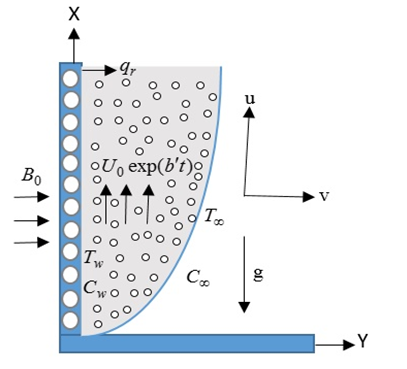Figure 1. Physical model and coordinate system

According to Bilal et al. , the extra stress tensor for the Prandtl fluid model is defined as follows:

$\tau =\frac{\text{A}\,\text{arcsin}\sqrt{\,\frac{1}{C}{{\left( \frac{\partial u}{\partial y} \right)}^{2}}+{{\left( \frac{\partial v}{\partial x} \right)}^{2}}}}{\sqrt{\,{{\left( \frac{\partial u}{\partial y} \right)}^{2}}+{{\left( \frac{\partial v}{\partial x} \right)}^{2}}}}\frac{\partial \bar{u}}{\partial y}$              (1)

where, A and C are material constants of Prandtl fluid model. Under these assumptions the dimensional continuity, momentum, energy and concentration equations are follows:

$\frac{\partial u}{\partial x}+\frac{\partial v}{\partial y}=0$               (2)

\begin{align} & \frac{\partial u}{\partial t}+u\frac{\partial u}{\partial x}+v\frac{\partial u}{\partial y}=\upsilon \frac{A}{C}\frac{{{\partial }^{2}}u}{\partial {{y}^{2}}}+ \\ & \upsilon \frac{A}{2{{C}^{3}}}{{\left( \frac{\partial u}{\partial y} \right)}^{2}}\frac{{{\partial }^{2}}u}{\partial {{y}^{2}}}+g{{\beta }_{T}}\left( T-{{T}_{\infty }} \right) \\ & +g{{\beta }_{C}}\left( C-{{C}_{\infty }} \right)-\frac{A}{C}\frac{\upsilon }{{{k}^{*}}}u-\frac{\sigma B_{0}^{2}u}{\rho } \\ \end{align}               (3)

$\frac{\partial T}{\partial t}+u\frac{\partial T}{\partial x}+v\frac{\partial T}{\partial y}=\frac{k}{\rho {{C}_{p}}}\frac{{{\partial }^{2}}T}{\partial {{y}^{2}}}-\frac{1}{\rho {{C}_{p}}}\frac{\partial {{q}_{r}}}{\partial y}+\frac{Q}{\rho {{C}_{p}}}\left( T-{{T}_{\infty }} \right)$                (4)

$\frac{\partial C}{\partial t}+u\frac{\partial C}{\partial x}+v\frac{\partial C}{\partial y}={{D}_{m}}\frac{{{\partial }^{2}}C}{\partial {{y}^{2}}}+\frac{{{D}_{m}}{{K}_{T}}}{{{T}_{m}}}\frac{{{\partial }^{2}}T}{\partial {{y}^{2}}}$                      (5)

The associate initial and boundary conditions according to the present problem are,

\left. \begin{align} & t\le 0,\,u=0,\,v=0,\,\,T={{T}_{w}},\,C={{C}_{w}}\,\,\,\,\,\text{for}\,\,\text{all}\,\,y \\ & t>0,\,u={{U}_{0}}\,\exp \left( b\frac{U_{0}^{2}}{\upsilon }t \right),\,v=0,\, \\ & T={{T}_{\infty }}+\left( {{T}_{w}}-{{T}_{\infty }} \right)\exp \left( \frac{U_{0}^{2}}{\upsilon }t \right)\,,\, \\ & C={{C}_{\infty }}+\left( {{C}_{w}}-{{C}_{\infty }}\, \right)\,\exp \left( \frac{U_{0}^{2}}{\upsilon }t \right)\, \\ & \,u=0,\,\,v=0,\,\,\,T\to {{T}_{\infty }},\,\,C\to {{C}_{\infty }}\,\,\,\,\,\,\,\text{as}\,\,y\to \infty \\ \end{align} \right\}               (6)

Since the solution of the governing equations under the initial and boundary conditions have been based on the finite difference method. It is to be required to make the equations dimensionless the following dimensionless variables are used.

\begin{align} & U=\frac{u}{{{U}_{0}}};\,\,V=\frac{v}{{{U}_{0}}};\,Y=\frac{y{{U}_{0}}}{\upsilon };\,\,X=\frac{x{{U}_{0}}}{\upsilon };\, \\ & \tau =\frac{tU_{0}^{2}}{\upsilon };\,\,T={{T}_{\infty }}+\bar{T}\left( {{T}_{w}}-{{T}_{\infty }} \right);\,\,\,C={{C}_{\infty }}+\bar{C}\left( {{C}_{w}}-{{C}_{\infty }} \right) \\ \end{align}                (7)

We obtained the following nonlinear coupled partial differential equations in terms of dimensionless variables by using above dimensionless variables and parameters,

$\frac{\partial U}{\partial X}+\frac{\partial V}{\partial Y}=0$                 (8)

$\frac{\partial U}{\partial \tau }+U\frac{\partial U}{\partial X}+V\frac{\partial U}{\partial Y}=\alpha \frac{{{\partial }^{2}}U}{\partial {{Y}^{2}}}+\beta {{\left( \frac{\partial U}{\partial Y} \right)}^{2}}\frac{{{\partial }^{2}}U}{\partial {{Y}^{2}}}+{{G}_{r}}\bar{T}+{{G}_{m}}\bar{C}-MU-\alpha {{K}_{p}}U$                     (9)

$\frac{\partial \bar{T}}{\partial \tau }+U\frac{\partial \bar{T}}{\partial X}+V\frac{\partial \bar{T}}{\partial Y}=\frac{1}{{{P}_{r}}}\left\{ \left( 1+\frac{4}{3}{{R}_{a}} \right)\frac{{{\partial }^{2}}\bar{T}}{\partial {{Y}^{2}}} \right\}+S\,\bar{T}$                   (10)

$\frac{\partial \bar{C}}{\partial \tau }+U\frac{\partial \bar{C}}{\partial X}+V\frac{\partial \bar{C}}{\partial Y}=\frac{1}{{{S}_{c}}}\frac{{{\partial }^{2}}\bar{C}}{\partial {{Y}^{2}}}+{{S}_{r}}\frac{{{\partial }^{2}}\bar{T}}{\partial {{Y}^{2}}}$           (11)

Also, the associate boundary conditions according to the present problem are,

\left. \begin{align} & \tau >0,\,\,\,\,\,U=\text{exp}\,(b\tau ),\,V=0,\,\bar{T}=\text{exp}\,(\tau ),\,\bar{C}=\text{exp}\,(\tau )\,\,\,\,\text{at}\,\,Y=0 \\ & \,\,\,\,\,\,\,\,\,\,\,\,\,\,\,\,\,U=0,\,\,\bar{T}\to 0,\,\bar{C}\to 0\,\,\,\,\,\,\,\,\,\,\,\,\,\,\,\,\,\,\,\,\,\,\,\,\,\,\,\,\,\,\,\,\,\,\,\,\,\,\,\,\,\,\,\,\,\,\,\,\,\,\,\,\,\,\,\,\text{as}\,\,Y\to \infty \\ \end{align}\right\}            (12)

where is the time constant, Prandlt number: ${{P}_{r}}=\frac{\upsilon \rho {{C}_{p}}}{k}$; Grashof number: ${{G}_{r}}=\frac{\upsilon g{{\beta }_{T}}({{T}_{w}}-{{T}_{\infty }})}{U_{0}^{3}}$; Heat source parameter: $S=\frac{Q\upsilon }{\rho {{C}_{p}}U_{0}^{2}}$; magnetic parameter: $\,M=\frac{\sigma B_{0}^{2}\upsilon }{\rho U_{0}^{2}}$; Schmidt number: ${{S}_{c}}=\frac{\upsilon }{{{D}_{m}}}$; Modified Grashof number: $\,{{G}_{m}}=\frac{\upsilon g{{\beta }_{c}}({{C}_{w}}-{{C}_{\infty }})}{U_{0}^{3}}$; Radiation parameter: ${{R}_{a}}=\frac{4{\sigma }'\,T_{\infty }^{3}}{{k}'k}$; Prandlt fluid parameter: $\alpha =\,\,\frac{1}{\mu AC}$; elastic parameter: $\beta =\frac{{{A}^{3}}}{2{{C}^{2}}\upsilon }$and Soret number: ${{S}_{r}}=\frac{{{D}_{m}}{{K}_{T}}}{{{T}_{m}}\upsilon }\frac{({{T}_{w}}-{{T}_{\infty }})}{({{C}_{w}}-{{C}_{\infty }})}$.

The skin friction, Nusselt number and Sherwood number are found respectively by the following with Non-dimensional form as:

${{C}_{f}}=-\frac{1}{2\sqrt{2}}{{({{G}_{r}})}^{-\frac{3}{4}}}{{\left( \frac{\partial U}{\partial Y} \right)}_{Y=0}};{{N}_{u}}=\frac{1}{\sqrt{2}}{{({{G}_{r}})}^{-\frac{3}{4}}}{{\left( \frac{\partial \bar{T}}{\partial Y} \right)}_{Y=0}};{{S}_{h}}=\frac{1}{\sqrt{2}}{{({{G}_{r}})}^{-\frac{3}{4}}}{{\left( \frac{\partial \bar{C}}{\partial Y} \right)}_{Y=0}}$                   (13)

Three dimensional stream functions exist physically but cannot be represented mathematically. So the Stream function $\psi(X, Y)$ can be exit two dimensionally with the velocity components as:

$U=\frac{\partial \psi }{\partial Y},\,V=-\frac{\partial \psi }{\partial X}$                (14)

3. Calculation Technique

The region of the flow is divided into a grid or mesh of lines parallel to X and Y axis where X- axis is taken along the plate and Y-axis is normal to the plate. It is considered that the plate of height Xmax(=125) i.e. X varies from 0 to 125 and regard Ymax(=125) i.e. Y varies from 0 to 25. There are m=150 and n=300 grid spacing in the X and Y directions respectively as shown in Figure 2. It is assumed that $\Delta X, \Delta Y$ are constant mesh sizes along $X$ and $Y$ directions respectively and taken, $\Delta X=0.83(0 \leq X \leq 125) ; \Delta Y=0.83(0 \leq \mathrm{Y} \leq 125) ;$ With the smaller time-step, $\Delta \tau=0.0005$ and ${U}',\,\,{\bar{T}}'\,\,\text{and}\,\,{\bar{C}}'$ denote the values of $U,\bar{T}\,\text{and}\,\,\,\bar{C}$ at the end of a time-step respectively.

## 2.pngFigure 2. The finite difference space grid

By using explicit finite difference method (EFDM) the following equations are obtained respectively as:

$\frac{{{U}_{i,j}}-{{U}_{i-1,j}}}{\Delta X}+\frac{{{V}_{i,j}}-{{V}_{i,j-1}}}{\Delta Y}=0$    (15)

\begin{align} & \frac{{{{{U}'}}_{i,j}}-{{U}_{i,j}}}{\Delta \tau }+{{U}_{i,j}}\left( \frac{{{U}_{i,j}}-{{U}_{i-1,j}}}{\Delta X} \right)+ \\ & {{V}_{i,j}}\left( \frac{{{U}_{i,j+1}}-{{U}_{i,j}}}{\Delta \Upsilon } \right)=\alpha \left( \frac{{{U}_{i,j+1}}-2{{U}_{i,j}}+{{U}_{i,j-1}}}{{{\left( \Delta \Upsilon \right)}^{2}}} \right)+{{G}_{r}}{{{\bar{T}}}_{i,j}} \\ \end{align}

\begin{align} & \,\beta {{\left( \frac{{{U}_{i,j+1}}-{{U}_{i,j}}}{\Delta \Upsilon } \right)}^{2}}\left( \frac{{{U}_{i,j+1}}-2{{U}_{i,j}}+{{U}_{i,j-1}}}{{{\left( \Delta \Upsilon \right)}^{2}}} \right) \\ & +{{G}_{m}}{{{\bar{C}}}_{i,j}}-M{{U}_{i,j}}-\alpha {{k}_{p}}{{U}_{i,j}} \\ \end{align}                 (16)

\begin{align} & \frac{{{{{\bar{T}}'}}_{i,j}}-{{{\bar{T}}}_{i,j}}}{\Delta \tau }+{{U}_{i,\,j}}\frac{{{{\bar{T}}}_{i,j}}-{{{\bar{T}}}_{i-1,j}}}{\Delta X}+{{V}_{i,\,j}}\frac{{{{\bar{T}}}_{i,j+1}}-{{{\bar{T}}}_{i,j}}}{\Delta Y} \\ & =\frac{1}{{{P}_{r}}}\left( 1+\frac{4}{3}{{R}_{a}} \right)\frac{{{{\bar{T}}}_{i,j+1}}-2{{{\bar{T}}}_{i,j}}+{{{\bar{T}}}_{i,j-1}}}{{{\left( \Delta Y \right)}^{2}}}+S\,{{{\bar{T}}}_{i,j}}\, \\ \end{align}              (17)

\begin{align} & \frac{{{{{\bar{C}}'}}_{i,j}}-{{C}_{i,j}}}{\Delta \tau }+{{U}_{i,\,j}}\frac{{{{\bar{C}}}_{i,j}}-{{{\bar{C}}}_{i-1,j}}}{\Delta X}+{{V}_{i,\,j}}\frac{{{{\bar{C}}}_{i,j+1}}-{{{\bar{C}}}_{i,j}}}{\Delta Y} \\ & =\left( \frac{1}{{{S}_{c}}} \right)\frac{{{{\bar{C}}}_{i,j+1}}-2{{{\bar{C}}}_{i,j}}+{{{\bar{C}}}_{i,j-1}}}{{{\left( \Delta Y \right)}^{2}}}\,+{{S}_{r}}\frac{{{{\bar{T}}}_{i,j+1}}-2{{{\bar{T}}}_{i,j}}+{{{\bar{T}}}_{i,j-1}}}{{{\left( \Delta Y \right)}^{2}}} \\ \end{align}                    (18)

In this case, the associate boundary conditions according to the present problem are,

\left. \begin{align} & \tau >0,\,\,\,\,\,U_{i,\,0}^{n}=\text{exp}\,(bn\Delta \tau ),\,\,V_{i,\,0}^{n}=0,\,\bar{T}_{i,\,0}^{n}=\text{exp}\,(n\Delta \tau ),\,\, \\ & \bar{C}_{i,\,0}^{n}=\text{exp}\,(n\Delta \tau )\,\,\,\,\,\,\,\,\,\,\text{at}\,\,\text{Y}=\text{0} \\ & \,\,U_{i,\,L}^{n}=0,\,\,\,\bar{T}_{i,\,L}^{n}\to 0,\,\,\bar{C}_{i,\,L}^{n}\to 0\,\,\text{where}\,\,L\to \infty \\ \end{align} \right\}                   (19)

4. Stability and Convergence Analysis

The stability conditions for the present problem are:

$\alpha \frac{2\Delta \tau }{{{(\Delta Y)}^{2}}}+U\frac{\Delta \tau }{\Delta X}+\left| -V \right|\,\frac{\Delta \tau }{\Delta Y}+\frac{{{K}_{p}}\Delta \tau }{2}\le 1$          (20)

${{R}_{a}}\frac{2\Delta \tau }{{{(\Delta Y)}^{2}}}+\frac{1}{{{P}_{r}}}\frac{2\Delta \tau }{{{(\Delta Y)}^{2}}}+U\frac{\Delta \tau }{\Delta X}+\left| -V \right|\,\frac{\Delta \tau }{\Delta Y}-\frac{S\,\Delta \tau }{2}\le 1$            (21)

$\frac{1}{{{S}_{c}}}\frac{2\Delta \tau }{{{(\Delta Y)}^{2}}}+U\frac{\Delta \tau }{\Delta X}+\left| -V \right|\,\frac{\Delta \tau }{\Delta Y}\le 1$               (22)

Since the initial condition, U=V=0 at t=0. So, the convergence criteria of the present problem are Sc³ 0.15, Pr ³ 0.62 and Le³0.87 respectively.

5. Results and Discussion

To obtain the results of the present problem an explicit finite difference method (EFDM) has been used and the approximate solutions are obtained for various parameters for the purpose of result and discussing. The physical situation of velocity, temperature, concentration, skin friction, Nusselt number, Sherwood number, streamlines and isotherms for vertical plate within the boundary conditions are obtained for the default parameters which are chosen as: M=1.0, Pr=0.63, Sc=0.22, b=0.01, S=0.1, a=0.01, Sr=1, Gm=5, Gr=5, Kp=0.5, and Ra=1.0 with time t=1.

The outcome of different values of the elastic parameter (b), Prandlt fluid parameter (a), magnetic parameter (M), permeability of porous medium (Kp), Schmidt number (Sc) and Prandtl number (Pr) on velocity profiles are displayed in the Figure 3(a) and Figure 3(b). It is observed that velocity profiles are increasing as elastic parameter (b) and Prandlt fluid parameter (a) but decreasing due to the magnetic parameter (M), permeability of porous medium (Kp), Schmidt number (Sc) and Prandtl number (Pr). Physically, the magnetic parameter (M) and permeability of porous medium (Kp) which increases a resistive force. For this resistive force, velocity profiles are decreasing with the increasing of magnetic parameter (M) and permeability of porous medium (Kp).

## 3.png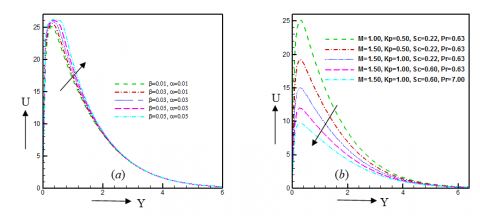Figure 3. Illustration of (a) Velocity profiles for different values of b and a (b) Velocity profiles for different values of M, Kp, Sc and Pr

## 4.pngFigure 4. Illustration of (a) Temperature profiles for different values of Pr and (b) Temperature profiles for different values of S and Ra

## 5.png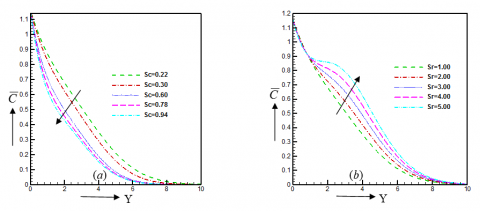Figure 5. Illustration of (a) Concentration profiles for different values of Sc and (b) Concentration profiles for different values of Sr

From Figure 4(a) and Figure 4(b), it is notified that temperature profiles are decreasing due to the increase of Prandtl number but increasing due to the increases of radiation parameter and heat source parameter. The main things of such mechanism on temperature profiles as heat source parameter produced energy in the fluid flow which increases the temperature profiles. Also, temperature profiles are increasing for differences values of radiation parameter. Physically, the radiation parameter provides more heat into the fluid, which leads to increases in the thermal boundary layer thickness.

The impact of Schmidt number and Soret number on concentration profiles are displayed in Figure 5(a) and Figure 5(b). Schmidt number (Sc) is a dimensionless number which decrease the concentration profiles. It is true by physically that the Schmidt number increases, the density of the fluid as well as concentration buoyancy force in the fluid. Also, the concentration boundary layer thickness increases consequently for the increasing of Soret number which leads the concentration profiles.

## 6.png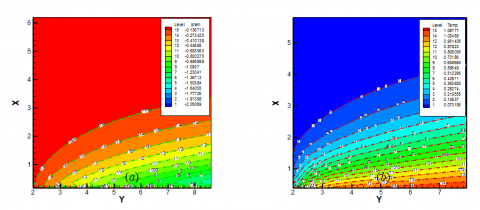Figure 6. Illustration of (a) Streamlines for magnetic parameter M =0.50 to M=1.50 and (b) Isotherms for magnetic parameter M =0.50 to M=1.50

## 7.png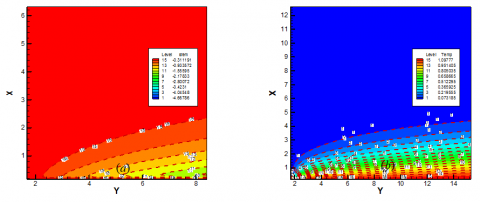Figure 7. Illustration of (a) Streamlines for Prandlt fluid parameter a=0.01 to a=0.03and (b) Isotherms for Prandlt fluid parameter a=0.01 to a=0.03

## 8.png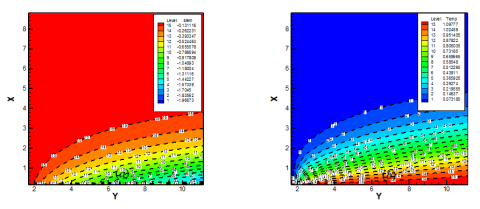Figure 8. Illustration of (a) Streamlines for radiation parameter Ra=1.0 to Ra=1.50 and (b) Isotherms for radiation parameter Ra=1.0 to Ra=1.50

The impact of magnetic parameter (M) on streamlines and isotherms are represented in Figure 6(a) and Figure 6(b). The momentum boundary layer thickness increases and thermal boundary layer thickness decreases due to increase of magnetic parameter M=0.50 to M=1.50. Also, the effect of Prandlt fluid parameter (a) on streamlines and isotherms are represented in Figure 7(a) and Figure 7(b). Here, we observed that momentum boundary layer thickness and thermal boundary layer thickness both are increasing due to the increase of Prandlt fluid parameter from a=0.01 to a=0.03. Further, the influence of radiation parameter (Ra) on streamlines and isotherms are represented in Figure 8(a) and Figure 8(b). Here, we observed that momentum boundary layer thickness and thermal boundary layer thickness both are decreasing due to the increase of radiation parameter (Ra) from Ra=1.0 to Ra=1.50.

Table 1 represents the numerical values of different parameters such as permeability of porous medium, elastic parameter, radiation parameter, Schmidt number, Prandlt fluid parameter, heat source parameter, Soret number and magnetic parameter on skin friction (Cf), Nusselt number(Nu) and Sherwood number (Sh). It is showed that the skin friction increases with elastic parameter, radiation parameter, heat source parameter and magnetic parameter but decreases qualitatively with an increase of permeability of porous medium, Schmidt number, Prandlt fluid parameter and Soret number. On the other hand, the Nusselt number is an increasing function of permeability of Prandlt fluid parameter and Soret number whereas this tendency is quite opposite for permeability of porous medium, elastic parameter, radiation parameter, Schmidt number, heat source parameter and magnetic parameter. Also it is noticed that the Sherwood number increases with the increasing of elastic parameter, radiation parameter, Schmidt number, Prandlt fluid parameter, heat source parameter, Soret number and magnetic parameter radiation parameter Lewis number but decreases qualitatively just with the increasing of permeability of porous medium, radiation parameter, Schmidt number, Prandlt fluid parameter, heat source parameter, Soret number and magnetic parameter.

Table 1. Variation of different parameters on local skin friction, Nusselt number and Sherwood number for Prandtl nanofluid.

 Kp b Ra Sc a S Sr M Cf Nu Sh 0.50 1.00 1.50 0.01 0.01 0.01 0.50 0.50 0.50 0.22 0.22 0.22 0.01 0.01 0.01 0.10 0.10 0.10 1.00 1.00 1.00 1.00 1.00 1.00 -0.07367 -0.08273 -0.10372 0.98203 0.79920 0.30980 0.59098 0.40328  0.25673 0.50 0.50 0.50 0.01 0.03 0.05 0.50 0.50 0.50 0.22 0.22 0.22 0.01 0.01 0.01 0.10 0.10 0.10 1.00 1.00 1.00 1.00 1.00 1.00 -0.04783 -0.05820 -0.08394 0.49023 0.47655 0.46992 0.50023 0.54773 0.57373 0.50 0.50 0.50 0.01 0.01 0.01 0.50 1.50 2.50 0.22 0.22 0.22 0.01 0.01 0.01 0.10 0.10 0.10 1.00 1.00 1.00 1.00 1.00 1.00 -0.03040 0.01928 0.04555 0.50983 0.49797 0.28973 0.48763 0.37653 0.36033 0.50 0.50 0.50 0.01 0.01 0.01 0.50 0.50 0.50 0.22 0.30 0.60 0.01 0.01 0.01 0.10 0.10 0.10 1.00 1.00 1.00 1.00 1.00 1.00 0.93723 0.83022 0.73996 0.47634 0.39874 0.27653 0.10237 0.08676  0.07897 0.50 0.50 0.50 0.01 0.01 0.01 0.50 0.50 0.50 0.22 0.22 0.22 0.01 0.02 0.03 0.10 0.10 0.10 1.00 1.00 1.00 1.00 1.00 1.00 0.27302 0.19343 0.14903 0.29034 0.37845 0.56742 0.34286 0.21661 0.19567 0.50 0.50 0.50 0.01 0.01 0.01 0.50 0.50 0.50 0.22 0.22 0.22 0.01 0.01 0.01 0.10 0.50 1.00 1.00 1.00 1.00 1.00 1.00 1.00 0.03891 0.07922 0.09039 0.42792 0.20554 0.18443 0.38788 0.28768 0.19223 0.50 0.50 0.50 0.01 0.01 0.01 0.50 0.50 0.50 0.22 0.22 0.22 0.01 0.01 0.01 0.10 0.10 0.10 1.00 2.00 2.00 1.00 1.00 1.00 0.98820 0.86829 0.58929 0.47662 0.58778 0.76273 0.39879 0.20199 0.10682 0.50 0.50 0.50 0.01 0.01 0.01 0.50 0.50 0.50 0.22 0.22 0.22 0.01 0.01 0.01 0.10 0.10 0.10 1.00 1.00 1.00 1.00 1.50 2.00 0.78829 0.89830 0.93068 0.38648  0.27809 0.25092 0.48772 0.20217 0.18982
6. Conclusion

From the present numerical investigation, the following conclusions have been drawn:

(1) Velocity profiles are increasing respectively as Prandlt fluid parameter.

(2)  Skin friction increases with the increase of elastic parameter, radiation parameter, heat source parameter and magnetic parameter but decreases qualitatively with an increase of permeability of porous medium, Schmidt number,  Prandlt fluid parameter and Soret number.

(3)  It is observed that permeability of porous medium and magnetic parameter decreases the velocity distribution.

(4) Radiation parameter increases the velocity and temperature profiles.

(5)  Momentum boundary layer thickness and thermal boundary layer thickness both are decreasing due to the increase of radiation parameter.

(6) Temperature profiles are increasing due to increases of radiation parameter and heat source parameter but decrease due to the increasing of Prandtl number.

References

 Akbar, N.S. (2014). Blood flow analysis of Prandtl fluid model in tapered stenosis arteries. Ain Shams Engineering Journal, 5(4): 1267-1275. https://doi.org/10.1016/j.asej.2014.04.014

 Nadeema, S., Haqa, R.U., Lee, C. (2012). MHD flow of a Casson fluid over an exponentially shrinking sheet. Scientia Iranica, 19(6): 1550-1553. https://doi.org/10.1016/j.scient.2012.10.021

 Bilal, S., Rehman, K.U., Malik, M.Y., Hussain, A., Awais, M. (2017). Effect logs of double diffusion on MHD Prandtl nano fluid adjacent to 4 stretching surface by way of numerical approach. Results in Physics, 7: 470–479. https://doi.org/10.1016/j.rinp.2016.11.008

 Nadeem, S., Ijaz, S., Akbar, N.S. (2013). Nanoparticle analysis for blood flow of Prandtl fluid model with stenosis. International Nano Letters, 3(1): 35. https://doi.org/10.1186/2228-5326-3-35

 Choi, S. (1995). Enhancing thermal conductivity of fluids with nanoparticles. ASME-Publ. Fed, 231: 99-106.

 Ahmmed, S.F., Biswas, R. (2019). Effects of radiation and chemical reaction on MHD unsteady heat and mass transfer of nanofluid flow through a vertical plate. Modelling Measurement and Control B, 87(4): 213-220.

 Hossain, M.D., Samad, M.A., Alam, M.M. (2016). MHD Free Convection and Mass Transfer Flow through a Vertical Oscillatory Porous Plate in a Rotating Porous Medium with Hall, Ion-Slip Currents and Heat Source. Measurement and Control B, 85(1): 28-42.

 Biswas, R., Mondal, M., Islam, A. (2019). A steady MHD natural convection heat transfer fluid flow through a vertical surface in the existence of Hall current and radiation. Instrumentation. Mesure, Métrologie, 17(2): 331-356. https://doi.org/10.3166/i2m.17.331-356

 Biswas, R., Hasan, M., Mondal, M., Shanchia, M.K., Bulbul, F., Ahmmed, S.F. (2019). A numerical superintendence with stability exploration of casson nanofluid flow in the effects of variable thermal conductivity and radiation. Advanced Science, Engineering and Medicine, 11(8): 697-707. https://doi.org/10.1166/asem.2019.2413

 Biswas, R., Mondal, M., Sarkar, D.R., Ahmmed, S.F. (2017). Effects of radiation and chemical reaction on MHD unsteady heat and mass transfer of Casson fluid flow past a vertical plate. Journal of Advances in Mathematics and Computer Science, 23(2): 1-16. https://doi.org/10.9734/JAMCS/2017/34292

 Biswas, R., Ahmmed, S.F. (2018). Effects of hall current and chemical reaction on magnetohydrodynamics unsteady heat and mass transfer of casson nanofluid flow through a vertical plate. Journal of Heat Transfer, 140(9): 092402. https://doi.org/10.1115/1.4039909

 Ahmmed, S.F., Biswas, R., Afikuzzaman, M. (2018). Unsteady magnetohydrodynamic free convection flow of nanofluid through an exponentially accelerated inclined plate embedded in a porous medium with variable thermal conductivity in the presence of radiation. Journal of Nanofluids, 7(5), 891-901. https://doi.org/10.1166/jon.2018.1520

 Afikuzzaman, M., Ferdows, M., Alam, M.M. (2015). Unsteady MHD casson fluid flow through a parallel plate with hall current. Procedia Engineering, 105, 287-293. https://doi.org/10.1016/j.proeng.2015.05.111

 Afikuzzaman, M., Biswas, R., Mondal, M., Ahmmed, S. (2018). MHD free convection and heat transfer flow through a vertical porous plate in the presence of chemical reaction. Frontiers in Heat and Mass Transfer (FHMT), 11: 13. http://dx.doi.org/10.5098/hmt.11.13

 Khalid, A., Khan, I., Khan, A., Shafie, S. (2016). Unsteady MHD free convection flow of Casson fluid past over an oscillating vertical plate embedded in a porous medium. Engineering Science and Technology, 18(3): 309-317. https://doi.org/10.1016/j.jestch.2014.12.006

 Tripathy, R.S., Dash, G.C., Mishra, S.R., Baag, S. (2015). Chemical reaction effect on MHD free convective surface over a moving vertical plate through porous medium. Alexandria Engineering Journal, 54(3): 673-679. https://doi.org/10.1016/j.aej.2015.04.012

 Mondal, M., Biswas, R., Shanchia, K., Hasan, M., Ahmmed, S. F. (2019). Numerical Investigation with Stability Convergence Analysis of Chemically Hydromagnetic Casson Nanofluid Flow in the Effects of Thermophoresis and Brownian Motion. International journal of heat and technology, 37(1): 59-70. https://doi.org/10.18280/ijht.370107

 Biswas, R., Mondal, M., Shanchia, K., Ahmed, R., Abdus Samad, S.K., Ahmmed S.F. (2019). Explicit finite difference analysis of an unsteady magnetohydrodynamics heat and mass transfer micropolar fluid flow in the presence of radiation and chemical reaction through a vertical porous plate. Journal of nanofluids, 8(7): 1583-1591. https://doi.org/10.1166/jon.2019.1704

 Biswas, R., Mondal, M., Hossain, S., Urmi, K.F., Suma, U.K., Katun, M. (2019). A numerical investigation with hydromagnetic stability convergence analysis on unsteady heat and mass transfer fluid flow through a vertical porous plate. Advanced Science, Engineering and Medicine, 11(6): 687-696. https://doi.org/10.1166/asem.2019.2411

 Lavanya, B., Ratnam, A.L. (2019). Dufour and Soret effects on steady MHD free Convective flow past a vertical porous plate embedded in a porous medium with chemical reaction, radiation, heat generation and viscous dissipation. Advances in Applied Science and Research, 5(1): 127-142.